Problem 1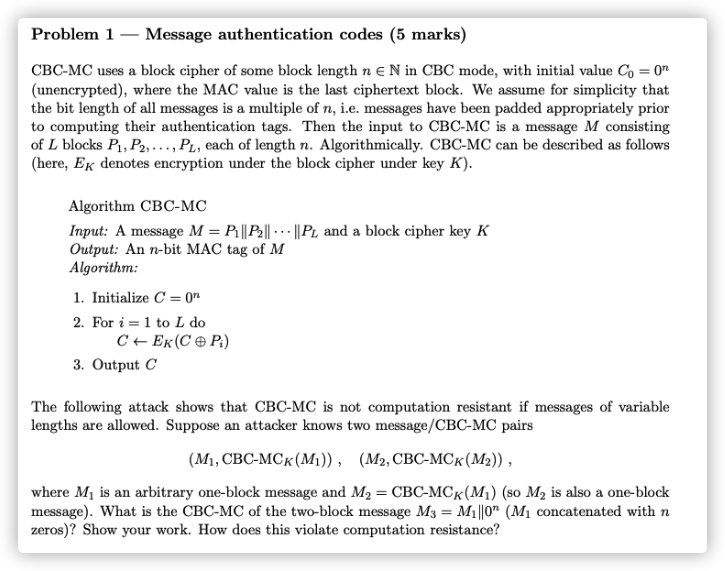According to the encryption algorithm,we can get the ciphertext of $M_3$ as follow：

\begin{aligned} \begin{array}{l} C_1=CBC-MC_k(0^n\oplus M_1)=M_2\\ C_3=CBC-MC_k(C_1\oplus0^n)=CBC-MC_k(M_2) \end{array} \end{aligned}

It cannot play a role in increasing the difficulty of calculation because $M_3$ is padded using $0^n$.

Problem 2a.

According to the question，we get the information as follow:$e_Ad_A=1(mod\ p-1),e_Bd_B=1(mod\ p-1)$

We can compute $M_A^{d_B}$ as the next process:

$M_A^{d_B}=(C_B^{d_A})^{d_B}=C_B^{d_Ad_B}=(C_A^{e_B})^{d_Ad_B}=C_A^{d_A}=M^{e_Ad_A}=M(modp)$

b.

Euler Theorem

c.

• $e_B$ will be computed when Eve hijacked the message $C_A$ and $C_B$ on the public communication channel. Then ,Eve will get $d_B$ according to $e_Bd_B=1(mod\ p-1)$.
• $d_A$ will be computed when Eve hijacked the message $C_B$ and $M_A$ on the public communication channel. Then ,Eve will get $e_A$ according to $e_Ad_A=1(mod\ p-1)$.

d.

The secret key is more shorter.

e.

The length of the plaintext of this algorithm has an upper bound.

Problem 3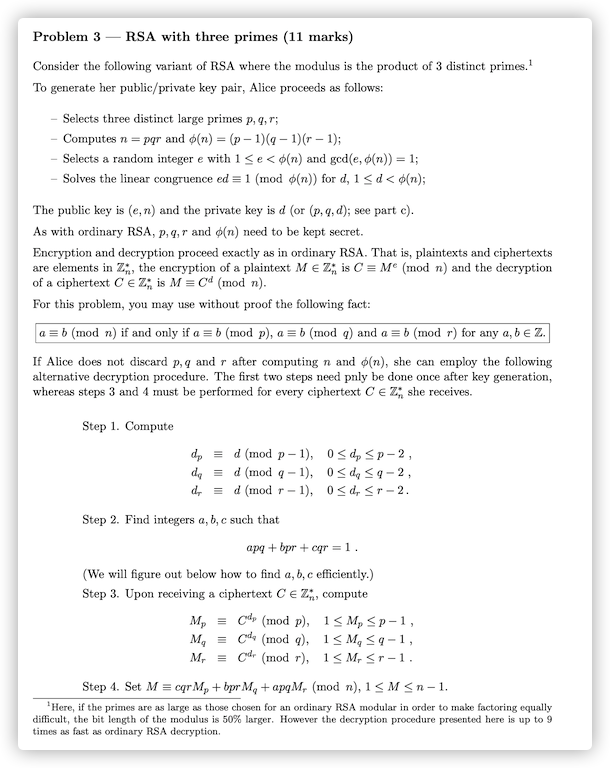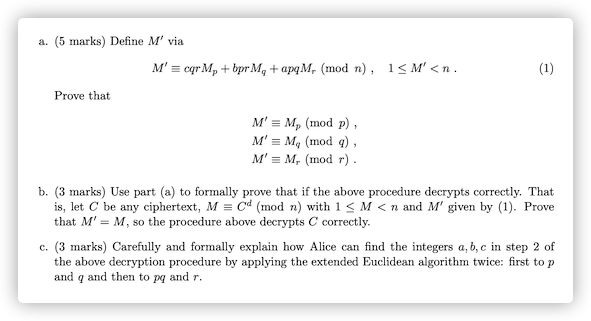a.

According to $cqr=1-bpr-apq$, both sides of equation (1) take modulo $p$ at the same time:

\begin{aligned} \begin{array}{l} M^{'}(modp)&=(cqrM_p+bprM_q+apqM_r+kn)(modp)\\ &=(cqrM_p+bprM_q+apqM_r+kpqr)(modp)\\ &=cqrM_p(modp)\\ &=(1-bpr-apq)M_p(modp)\\ &=M_p(modp) \end{array} \end{aligned}

Similarly, we can prove $M'\equiv M_q(modq)$ and $M'\equiv M_r(modr)$.

b.

According to Euler’s theorem:$M(modp)=C^d(modp)=C^{d(mod\varphi(p))}(modp)=C^{d_p}(modp)=M_p(modp)$.

Similarly, we can get $M(modq)=M_q(modq)$ and $M(modr)=M_r(modr)$.

Those result are as follows：

\begin{aligned} \left\{ \begin{array}{l} M\equiv M_p(mod\ p)\\ M\equiv M_q(mod\ q)\\ M\equiv M_r(mod\ r)\\ \end{array} \right. \end{aligned}

Let $n_p=n/p=qr,n_q=n/q=pr,n_r=n/r=pq$. Combining with $apq+bpr+cqr=1$,we can get $n_p^{-1}=c(modp),n_q^{-1}=b(modq),n_r^{-1}=a(modr)$.

To sum up，combining with the Chinese Remainder Theorem, we can see：

\begin{aligned} \begin{array}{l} M&=M_pn_pn_p^{-1}+M_qn_qn_q^{-1}+M_rn_rn_r^{-1} (modn)\\ &=M_p(qr)c+M_q(pr)b+M_r(pq)a(modn)\\ &=cqrM_p+bprM_q+apqM_r(modn) \end{array} \end{aligned}

That is, the above encryption is correct because we can get the proof of $M^{'}=M$.

c.

• According to $gcd(p,q)=1$$\exists x,y,s.t.xp+yq=1$
• Easy to know $gcd(pq,r)=1$$\exists s,t,\ s.t.s(pq)+tr=1$

We do the following calculations:

\begin{aligned} \begin{array}{l} 1&=s(pq)+tr\\ &=s(pq)+tr(xp+yq)\\ &=s(pq)+tx(pr)+ty(qr) \end{array} \end{aligned}

Then,$a=s,b=tx,c=ty$ are the integers.

Problem 4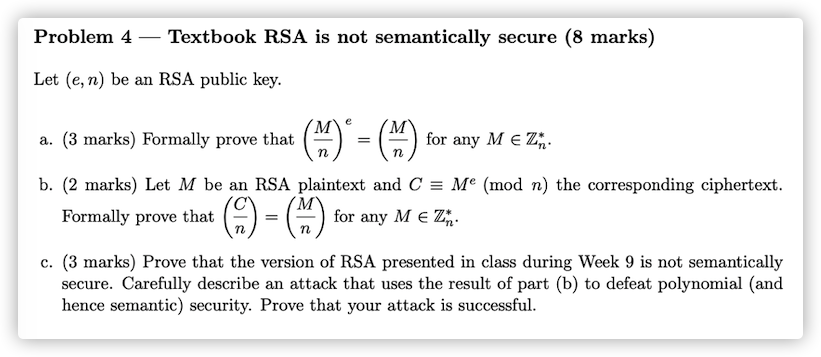a：

According to the Jacobi symbol:

$n=pq,\rightarrow \left(\frac{M}{n}\right)=\left(\frac{M}{p}\right)\left(\frac{M}{q}\right)$

Because of $gcd(e,\varphi(n))=1$,we can infer that $e$ is prime.

From the fact that both $p$ and $q$ are odd prime numbers:

• If $M|q$ or $M|p$, we can get ($0^e=0$), so the original formula clearly holds。
• If $\left(\frac{M}{p}\right)=-1,\left(\frac{M}{q}\right)=-1$,the original formula holds because of $[(-1)(-1)]^e=[(-1)(-1)]$.
• If $\left(\frac{M}{p}\right)=1,\left(\frac{M}{q}\right)=-1$,the original formula holds because of$[(1)(-1)]^e=[(1)(-1)]$.
• If $\left(\frac{M}{p}\right)=-1,\left(\frac{M}{q}\right)=1$,the original formula holds because of$[(-1)(1)]^e=[(-1)(1)]$.
• If $\left(\frac{M}{p}\right)=1,\left(\frac{M}{q}\right)=1$,the original formula holds because of$[(1)(1)]^e=[(1)(1)]$.

b:

According to $C=M^e+kn$, we can prove as follow combining with the conclusion of (a)：

$\left(\frac{C}{n}\right)=\left(\frac{M^e+kn}{n}\right)=\left(\frac{M^e}{n}\right)=\left(\frac{M}{n}\right)^e=\left(\frac{M}{n}\right)$

c:

RSA is not semantically secure：

Assuming we know the plain/ciphertext pairs,$(M_1,C_1),(M_2,C_2)$, then we can forge information by setting $M=M_1M_2$.

We can get the ciphertext of $M$ as follow:$C_M=(M_1M_2)^e=M_1^eM_2^e=C_1C_2(modn)$

Defeat polynomial security：

Suppose the adversary A randomly selects the quadratic non-residue $r$ and $m$ on modulo n：

• Let $M_0=rm,M_1=M$, and send them to the user.
• The user will select one of them and send its cipertext $M_b$ to A.
• A will compute $result=\left(\frac{M_b}{n}\right)$.If $result=-1$,$M_1$ is encrypted,otherwise $M_0$ is encrypted.

Proof as follows：

• If $M_0$ is encrypted，$M_b=M_0^e(modn)$.

According to the result of (b),A can compute $\left(\frac{M_b}{n}\right)=\left(\frac{rm}{n}\right)=\left(\frac{m}{n}\right)\left(\frac{r}{n}\right)=(-1)(-1)=1$

• If $M_1$ is encrypted,$M_b=M_1^e(modn)$.

According to the result of (b),A can compute$\left(\frac{M_b}{n}\right)=\left(\frac{m}{n}\right)=-1$

• Adversary A can distinguish the source of the encrypted message every time, which is a polynomial security attack.

Problem 5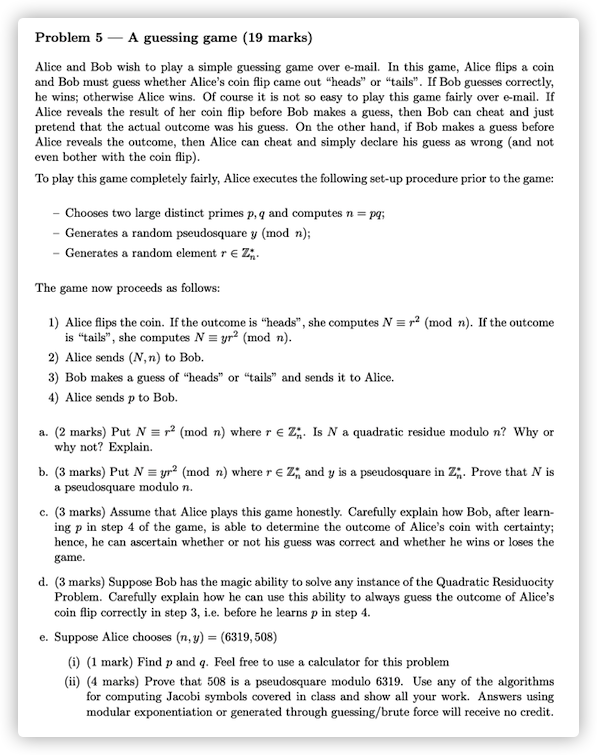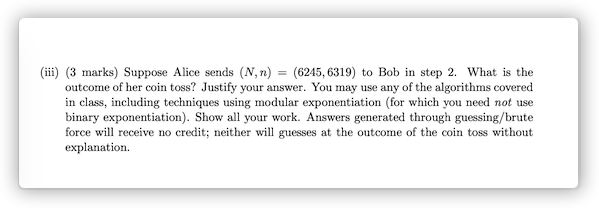a.

Yes,because $\exists r \in Z^*_n,s.t. N = r^2(modn)$

b.

Assuming $N=yr^2(modn)$ is a quadratic residue，$\exists t\in Z^*_n,s.t.t^2=yr^2(modn)$ will hold.

And then we can compute $y$ because $y=(tr^{-1})^2(modn)$. We can get the conclusion that $y$ is also a quadratic residue contrary to the statement of the question.

So $N$ is a pseudosquare modulo $n$.

c.

• Bob will compute $result=\left(\frac{N}{p}\right)$ after receiving the $p$.
• If $result=1$，the outcome of Alice’s coin is “heads", if $result=-1$,the outcome is ”tails“.

d.

Determine whether $N$ is a quadratic residue modulo $n$.

If $N$ is a quadratic residue ,send “heads” to Alice,otherwise “tails”.

e.

1.p=71,q=89

$\left(\frac{508}{6319}\right)=\left(\frac{508}{71}\right)\left(\frac{508}{89}\right)=\left(\frac{11+71*7}{71}\right)\left(\frac{89*5+63}{89}\right)=\left(\frac{11}{71}\right)\left(\frac{63}{89}\right)$

Compute $(11/71)$ and (63/89) as follow:

$(11/71) = -(5/11) = -(1/5) = -1$

$(63/89) = (26/63) = (13/63) = (11/13) = (2/11) = -(1/11) = -1$

Because $\left(\frac{508}{6319}\right)=(-1)(-1)=1$, 508 is a pseudosquare modulo 6319.

$\left(\frac{6245}{6319}\right)=\left(\frac{6245}{71}\right)\left(\frac{6245}{89}\right)=\left(\frac{68}{71}\right)\left(\frac{15}{89}\right)$

Compute $(68/71)$ and $(15/89)$ as follow:

$(68/71) = (34/71) = (17/71) = (3/17) = (2/3) = -(1/3) = -1$

$(15/89) = (14/15) = (7/15) = -(1/7) = -1$

Because $\left(\frac{6245}{6319}\right)=(-1)(-1)$,the outcome of coin is “tails”.

Problem 6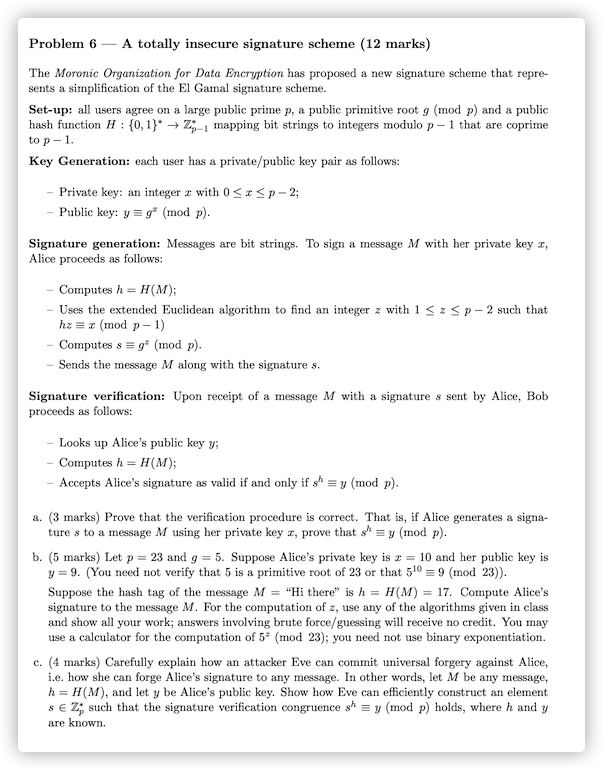a.

According to $hz=x(mod\varphi(p))$,

so $s^h=g^{hz}(modp)=g^x(modp)=y(modp)$

b.

Use the extend Euclidean algorithm to find the inverse of 17 modulo $\varphi(p)$

$1=5-2*2=7*5-2*17=7*22-9*17$

so $h^{-1}=(-9)(mod22)=13$.

According to $hz=x(mod(p-1))$

we can compute $z$ as $z=xh^{-1}(mod(p-1))=10*13mod22=20$

And then $s=g^z(modp)=5^{20}mod23=5^{23-1}*5^{-2}mod23=(25mod23)^{-1}(mod23)=12$

c.

$s=y^{-h}(modp)$

Problem 7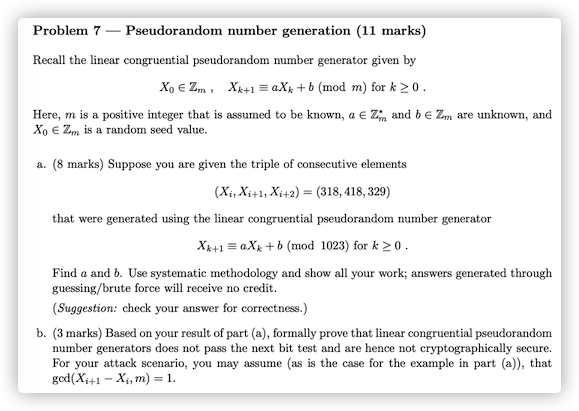a.

\begin{aligned} X_{i+1}&\equiv aX_{i}+b(mod\ m)\\ X_{i+2}&\equiv aX_{i+1}+b(mod\ m)\\ \end{aligned}

Easy to know：$X_{i+2}-X_{i+1}=a(X_{i+1}-X_i)(modm)$，so we can get the information：$-89=100a(mod1023)$

Use the extend Euclidean algorithm to find the inverse of 100 modulo 1023：

\begin{aligned} 1023&=100*10+23\\ 100&=23*4+8\\ 23&=8*2+7\\ 8&=7+1 \end{aligned}

\begin{aligned} 1&=8-7\\ &=8*3-23\\ &=100*3-23*13\\ &=100*133-1023*13 \end{aligned}

That’s mean $100^{-1}=133(mod1023)$.

$a=(-89)*100^{-1}(mod1023)=439(mod1023)$

According to $X_{i+1}= aX_{i}+b(mod\ m)$$b=418-439*318(mod1023)=967$

$aX_{i+1}+b=(439*418+967)(mod1023)=429=X_{i+2}$
• The sequence period $T$ is less than or equal to the modulo $m$.